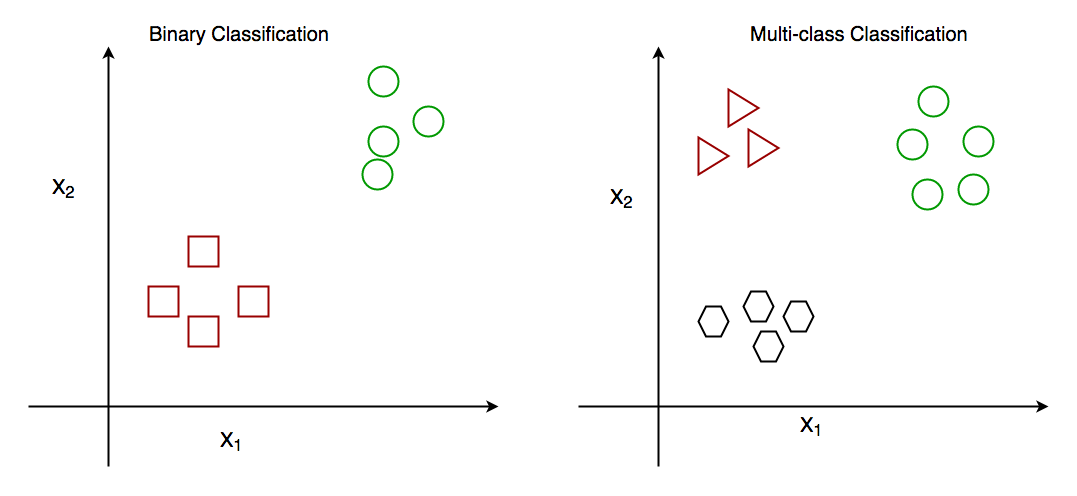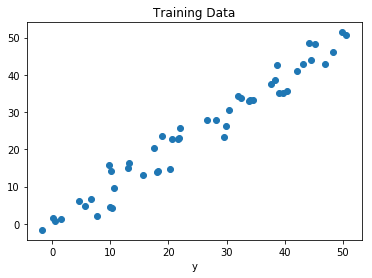GeeksforGeeks App
Open AppBrowser
Continue

# Classification vs Regression in Machine Learning

Classification and Regression are two major prediction problems that are usually dealt with in Data Mining and Machine Learning

## Classification Algorithms

Classification is the process of finding or discovering a model or function which helps in separating the data into multiple categorical classes i.e. discrete values. In classification, data is categorized under different labels according to some parameters given in the input and then the labels are predicted for the data.

• In a classification task, we are supposed to predict discrete target variables(class labels) using independent features.
• In the classification task, we are supposed to find a decision boundary that can separate the different classes in the target variable.

The derived mapping function could be demonstrated in the form of “IF-THEN” rules. The classification process deal with problems where the data can be divided into binary or multiple discrete labels. Let’s take an example, suppose we want to predict the possibility of the winning of a match by Team A on the basis of some parameters recorded earlier. Then there would be two labels Yes and No.Binary Classification and Multiclass Classification

### Types of Classification Algorithms

There are different types of State of the art classification algorithms that have been developed over time to give the best results for classification tasks by employing techniques like bagging and boosting.

## Regression Algorithms

Regression is the process of finding a model or function for distinguishing the data into continuous real values instead of using classes or discrete values. It can also identify the distribution movement depending on the historical data. Because a regression predictive model predicts a quantity, therefore, the skill of the model must be reported as an error in those predictions.

• In a regression task, we are supposed to predict a continuous target variable using independent features.
• In the regression tasks, we are faced with generally two types of problems linear and non-linear regression.

Let’s take a similar example in regression also, where we are finding the possibility of rain in some particular regions with the help of some parameters recorded earlier. Then there is a probability associated with the rain.Regression of Day vs Rainfall (in mm)

### Types of Regression Algorithms

There are different types of State of the art regression algorithms that have been developed over time to give the best results for regression tasks by employing techniques like bagging and boosting.

## Comparison between Classification and Regression

My Personal Notes arrow_drop_up